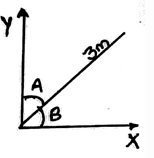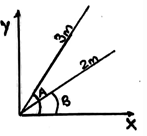# Test: Addition Of A System Of Coplanar Forces

## 14 Questions MCQ Test Engineering Mechanics | Test: Addition Of A System Of Coplanar Forces

Description
This mock test of Test: Addition Of A System Of Coplanar Forces for Mechanical Engineering helps you for every Mechanical Engineering entrance exam. This contains 14 Multiple Choice Questions for Mechanical Engineering Test: Addition Of A System Of Coplanar Forces (mcq) to study with solutions a complete question bank. The solved questions answers in this Test: Addition Of A System Of Coplanar Forces quiz give you a good mix of easy questions and tough questions. Mechanical Engineering students definitely take this Test: Addition Of A System Of Coplanar Forces exercise for a better result in the exam. You can find other Test: Addition Of A System Of Coplanar Forces extra questions, long questions & short questions for Mechanical Engineering on EduRev as well by searching above.
QUESTION: 1

### Which of the following statement is true?

Solution:

A scalar is any positive or negative physical quantity that can be completely specified by its magnitude. Examples of scalar quantities include length, mass, time, etc.

QUESTION: 2

### For two vectors defined by an arrow with a head and a tail. The length of each vector and the angle between them represents:

Solution:

For two vectors defined by an arrow with a head and a tail. The length of each vector and the angle between them represents their magnitude and direction of the line of action respectively. The head/tip of the arrow indicates the sense of direction of the vector.

QUESTION: 3

### If a vector is multiplied by a scalar:

Solution:

If a vector is multiplied by a scalar then its magnitude is increased by amount of that scalar’s magnitude. When multiplied by a negative scalar it going to change the directional sense of the vector.

QUESTION: 4

All the vectors quantities obey:

Solution:

All the vectors quantities obey parallelogram law of addition. Two vectors A and B (can be called as component vectors) are added to form a resultant vector. R = A+B.

QUESTION: 5

A force vector with magnitude R and making an angle α with the x-axis is having its component along x-axis and y-axis as:

Solution:

The component along x-axis is the cosine component of the vector. And the y-axis component of the vector is sine component, if the angle is being made with the x-axis. And 180- α for some of the trigonometric function may change their sign.

QUESTION: 6

Dividing the X-axis component and the Y-axis component of the of the vector making an angle with Y-axis α will give us.

Solution:

As the X-axis component of the vector becomes cos(90- α) and the Y-axis component becomes sin(90- α).Thus the division of both will give us Tan α.

QUESTION: 7

Vector shown in the figure below have a length of 3m and the angles shown A and B are 60 and 30 degrees each. Calculate the X-axis and Y-axis components:Solution:

The sine and the cosine components of the given vectors considering the angle B as the only angle of consideration comes 1.5m and 2.59m.

QUESTION: 8

Shown as in the figure below, A=60 degree and B=30 degree. Calculate the total length obtained by adding the x-axis component of both the vectors.Solution:

After getting the cosine components of the given vectors we obtain the total length of the x-axis components to be 3cos60 + 2cos30 = 3.23.

QUESTION: 9

The magnitude of the resultant of the two vectors is always_____________

Solution:

Yes, the magnitude of the resultant of the two vectors always depends on the angle between them. It might be greater or smaller than one of the vector’s length. For perfectly saying, it does depends upon the angle between them.

QUESTION: 10

If two equal vector forces are mutually perpendicular then the resultant force is acting at which angle as compared to one of the vector?

Solution:

The vectors are mutually perpendicular, this means that the angle between the forces is 90 degree. Thus the resultant will form at 45 degrees to any of the vector.

QUESTION: 11

What is the direction of the resultant vector if two vectors having equal length is placed in the Cartesian plane at origin as, one being parallel to and heading towards positive x-axis and the other making 165 degree with it and heading in the opposite direction of that of the first one?

Solution:

If one is heading towards positive X-axis and the other is in the other direction opposite to the first one, with both having same length and having an angle between them being obtuse, means that the direction is to be in the direction of either 1st quadrant or in 4nd quadrant.

QUESTION: 12

Force vector R is having a______________

Solution:

As it is a force vector, it means it is going to have a direction and a magnitude. The magnitude is not the square root of R, R is just the magnitude of the vector given.

QUESTION: 13

The resultant of three equal vectors having mutual angles being 120 degree and being originated from a single point is zero.

Solution:

As one can imagine three vectors coming out of a single point and are having a 120 degree angle with their adjacent ones, the resultant would be zero.

QUESTION: 14

Every point on the force vector is having the same magnitude and the same direction as the whole force vector have.

Solution:

The vector is made by joining the final and the starting point. If we consider any arbitrary point then the length would be calculated w.r.t the starting point, which might give different magnitude but same direction.